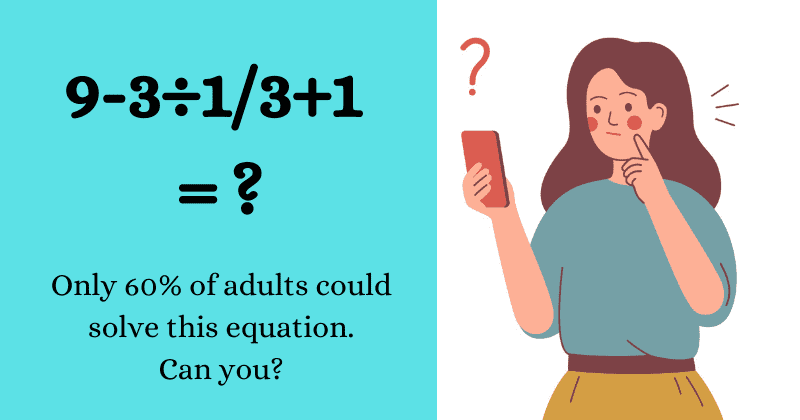Fun Puzzle: This Equation Has Baffled 40% Of Young Adults, Can You Solve It?

# Fun Puzzle: This Equation Has Baffled 40% Of Young Adults, Can You Solve It?

There is no unique story behind this mathematical equation, apart from the fact that the success rate of solving the equation decreased significantly.Not many can say they have loved doing maths in school but we often find ourselves taking on simple equations whenever they do go viral. Most of us will agree that the mathematical equations we solved in school didn't necessarily have any application in our day-to-day life. But it's important to note that the regular practice did help sharpen our young minds and kept us alert. However, those days of partaking in compulsory math quizzes are long gone and I for one have noticed significant atrophy in my maths skills compared to when I was in high school. While it doesn't bother me as much, it did rub people in Japan the wrong way especially when they noticed a decline in the accuracy rate of solving the same question by different batches.

A study published in Japan revealed that just 60% of adults, who were between the ages of 20 and 30, in the country were able to solve a particular maths question correctly. According to Soran News 24, there is no unique story or special meaning behind this mathematical equation, apart from the fact that the success rate of solving the equation decreased significantly since it was first introduced in the 1980s. At that time about 90% of the people of the same age group were able to solve the question correctly which is a stark contrast to the current 60%.

Everyone was left baffled by the report which revealed the gradual decrease in accuracy and understandably they couldn't help but wonder about the reason. It seems that the best way to get this answer is to try your hand at solving this particular problem. Take a look at the equation below:

We probably wouldn't have known about this mathematic adroitness crisis if it wasn't for a YouTube channel called MindYourDecisions. The channel released the video back in 2016 and it takes us through the process of solving it as well. But before we reveal the answer, why not try solving it first?

.

.

If you think the answer's 3, then I'm sorry to inform you that it is in fact the wrong answer. But I am hopeful that you'll get the right conclusion if you know the logic behind it. You may have been left with 3 if you subtracted 3 from 9 and divided the remainder by 3 and added 1 to it. If you've followed this method then you must have forgotten about the order of mathematical operations called PEDMAS. This particular sum is an excellent example of why you need to follow the rule to get the right answer.

What's interesting is the fact that most young adults in Japan, who got the equation wrong, did not make this mistake while answering the equation. Among the 40% of these people, most of them ended up with 9, and if you google the problem or enter it in your calculator the answer might confuse you a little. Turns out both Google and 40% of members in the group were not wrong because their intelligence decreased but it happened due to the way they read the equation.

This YouTube video explains how we see the problem as 3 divided by one-third however while entering it in our calculator, we type 3÷1/3. Due to this, the ÷ sign gets converted into a division slash and the equation becomes 3/1/3. Next, on solving 9-3/1/3+1 using PEDMAS we get 9. Click here if you wish to see all the steps of this form.

Now, to solve it correctly, you need to use brackets to ensure that the one-third fraction remains in the accurate form while solving. "30 years or so ago, it was generally understood that the '1/3' part of the problem wasn’t to be read as 'one-third,' but instead indicates that you need to divide," reports Soran News.

Here's how you need to construct the equation in order to get the accurate answer:

9-3÷1/3+1
9-3÷(1/3)+1
9-3×3+1
9-9+1
=1

Watch the video to know more:

References:

https://soranews24.com/2015/12/05/does-this-math-problem-prove-that-japans-20-somethings-are-getting-dumber/